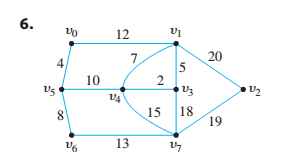Chapter 10.6, Problem 8ES### Discrete Mathematics With Applicat...

5th Edition
EPP + 1 other
ISBN: 9781337694193

#### Solutions

Chapter
Section### Discrete Mathematics With Applicat...

5th Edition
EPP + 1 other
ISBN: 9781337694193
Textbook Problem
1 views

# Use Prim’s algorithm starting with vertex a or v 0 to find a minimum spanning tree for each of the graphs in 7 and 8. Indicate the order in which edges are added to form each tree. 8. The graph of exercise 6.To determine

(a)

The minimum spanning tree for the given graph using Prim’s algorithm.

Explanation

Given information:

A weighted connected graph is given as,

Calculation:

Prim’s minimum cost spanning tree algorithm states that, one edge and one vertex are added at each step. The edge added is one of the least weight that connects the vertices already added in the spanning tree with those not in spanning tree, until we reach n1 edges where n is the number of vertices in the given graph.

When we apply Prim’s algorithm then, edges are added in the following order with a as start vertex

To determine

(b)

The minimum spanning tree for the given graph using Prim’s algorithm.

### Still sussing out bartleby?

Check out a sample textbook solution.

See a sample solution

#### The Solution to Your Study Problems

Bartleby provides explanations to thousands of textbook problems written by our experts, many with advanced degrees!

Get Started